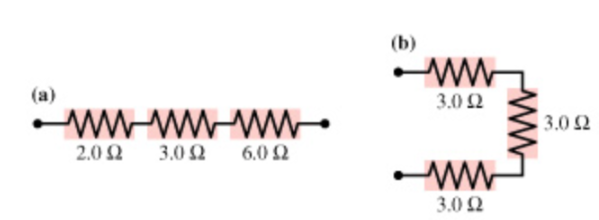# Problem: What is the equivalent resistance of group (a) of resistors shown in the figure?What is the equivalent resistance of group (b) of resistors shown in the figure?Express your answers using two significant figures.

###### FREE Expert Solution
95% (432 ratings)
###### FREE Expert Solution

Equivalent resistance,

For resistors in parallel,

$\overline{)\frac{\mathbf{1}}{{\mathbf{R}}_{\mathbf{eq}}}{\mathbf{=}}\frac{\mathbf{1}}{{\mathbf{R}}_{\mathbf{1}}}{\mathbf{+}}\frac{\mathbf{1}}{{\mathbf{R}}_{\mathbf{2}}}{\mathbf{+}}\frac{\mathbf{1}}{{\mathbf{R}}_{\mathbf{3}}}{\mathbf{.}}{\mathbf{.}}{\mathbf{.}}}$

For resistors in series,

$\overline{){{\mathbf{R}}}_{{\mathbf{eq}}}{\mathbf{=}}{{\mathbf{R}}}_{{\mathbf{1}}}{\mathbf{+}}{{\mathbf{R}}}_{{\mathbf{2}}}{\mathbf{+}}{{\mathbf{R}}}_{{\mathbf{3}}}{\mathbf{+}}{\mathbf{.}}{\mathbf{.}}{\mathbf{.}}}$

95% (432 ratings)###### Problem Details

What is the equivalent resistance of group (a) of resistors shown in the figure?
What is the equivalent resistance of group (b) of resistors shown in the figure?